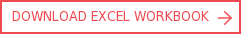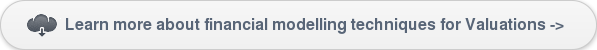View More Content
SHARE

Terminal value calculations in a financial modelCategoriesTutorialsTerminal value is the value of a project’s expected cash flow beyond the explicit forecast horizon. An estimate of terminal value is critical in financial modelling as it accounts for a large percentage of the project value in a discounted cash flow valuation. This tutorial focuses on ways in which terminal value can be calculated in a project finance model.

Net present value (NPV) can be used to calculate the value of a project/investment based on future cash flows. A firm or project potentially has infinite life. Its value, therefore, is the NPV of cash flows for an indefinite period into the futureHowever, forecasting results beyond certain periods is impractical and exposes such projections to a variety of risks. This limits their validity, as there is great uncertainty in predicting the project revenue or cost components, and industry or macroeconomic conditions beyond a few years.

Terminal value is a critical component to financial modelling for valuations and is often discussed in depth in our financial modelling courses.

To capture the value at the end of the forecasting period, a terminal value is included. Terminal value allows for the inclusion of the value of future cash flows beyond a several year projection period, while satisfactorily mitigating many of the problems of valuing such project cash flows.A high-quality estimate of terminal value is critical because it often accounts for a large percentage of the total value of the project in a discounted cash flow valuation. As a result, financial analysts and modellers should be familiar with the mechanics of terminal value, and how it is calculated, in order to ensure an accurate financial modelling and valuation exercise.

This tutorial with the accompanied Excel workbook illustrates various ways in which terminal value can be calculated.

Financial modelling calculations for terminal value estimations

Three methods will be discussed in this tutorial:

• Multiple earnings before interest, tax, depreciation and amortisation (EBITDA) approach
• Perpetuity approach
• Perpetuity with growth approach

In practice, academics tend to use the perpetuity growth model, while project financiers favour the exit multiple approach.

Ultimately, these methods are two different ways of saying the same thing. For both terminal value approaches it is essential to use a range of appropriate discount rates, the multiples and perpetuity growth rates in order to establish a functional valuation range.

Multiple EBITDA approach to assess terminal value

The multiple EBITDA approach measures the firm value of the enterprise – that is, the value of the business operations. In calculating enterprise value, only the operational value of the business is included. The formula to calculate the terminal value is:The present value (PV) of the terminal value is then added to the PV of the free cash flows in the projection period to arrive at an implied firm value.

A publicly-traded comparable company’s multiples are used in the calculation. This method is the easiest approach but, depending on the purposes of the valuation, the estimated EBITDA multiple may not provide an appropriate reference range.

There are some variations of multiple used in the terminal multiple approaches:

• P/E multiple
• Market to book multiple
• Price to revenue multiple
• Earnings before interest and tax (EBIT) multiple
• Multiple EBITDA approach

Calculating the terminal value based on perpetuity growth methodology

The perpetuity growth approach assumes that free cash flow will continue to grow at a constant rate into perpetuity. The terminal value can be estimated using this formula:What growth rate do we use when modelling? The constant growth rate is called a stable growth rate. While past growth is not always a reliable indicator of future growth, there is a correlation between current growth and future growth. A project currently growing at 10% probably has higher growth and a longer expected growth period than one now growing at five percent a year.

Using estimation of the growth rate in this approach makes it challenging, because inaccuracy in the assumption can provide an improper value. Therefore, analysts sometimes drop the growth rate in the formula to arrive at a more conservative terminal value.

Financial modelling of terminal value

For illustration on how the terminal value is estimated and used for valuation purpose in a project finance model, we have prepared an Excel workbook, which you can download at the top or bottom of this tutorial.

The example assumes that a project has 12-month constructions, and quarterly cash flow projections of 10 years. Three methods are demonstrated to estimate the terminal value. The assumptions are depicted in screenshot 1 below. In all methods, we need to establish the EBITDA or free cash flow at the last year of the projected period. The easiest way to do this is perhaps by constructing a simple binary (1, 0) flag.Screenshot 1: Financial modelling of a binary flag for end of operations

The terminal value calculation is very straightforward. Various methods of calculating the terminal value are shown below.Screenshot 2: Multiple EBITDA approach for Terminal Value calculations in a financial model

The final step is to add the terminal value into the project cash flow before calculating the NPV. In this example, it is assumed that the perpetuity approach is selected.

Terminal value modelling considerations

There are a few considerations in calculating terminal value in project finance modelling:

• Carefully establish the cash flow or EBITDA at the last year of the projected period. The usual mistake is to capture the cash flow falls in the last quarter/month, instead of summing up those in the last year of the projected period.
• Reminded to add the terminal value into the project cash flow before calculating the NPV.  As the project valuation does not stop at a terminal value calculation, remember to add the calculated terminal value into the project cash flow for NPV calculations.
• Select the appropriate multiple, growth rate and discount rate for the risk profile of the project, as it is a key variable in the terminal value calculation.
• Clearly show if the calculation is pre or post tax.Corality Training Academy - SMART CAMPUS

There are numerous other tutorials and examples related to financial modelling in Corality's SMART Campus. Some of the more popular items include the calculation of a DSRA, debt sizing vs debt sculpting and LLCR, which are all related to our suite of project finance modelling courses.

Additional related training courses include:

Financial Modelling Techniques for Valuation Analysis

LBO Transactions: Financial Modelling Techniques.by Rickard Wärnelid

Rickard's passion for financial modelling is built on specialist roles in the highly quantitative fields of derivatives and project finance, a career path complemented by an academic grounding in engineering physics. Born in Sweden and with global consulting and leadership experience, Rickard is an internationally recognised authority, speaker and thought-leader on the organisational benefits of best practice financial modelling.

view all